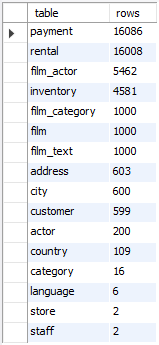## List tables by the number of rows in MySQL database

Article for:

This query returns a list of tables in a database (schema) with their number of rows.

## Notes

Some storage engines, such as MyISAM, store the exact count. For other storage engines, such as InnoDB, this value is an approximation, and may vary from the actual value by as much as 40% to 50%. In such cases, use SELECT COUNT(*) to obtain an accurate count.

## Query

``````select table_name as 'table',
table_rows as 'rows'
from information_schema.tables
where table_schema = 'your database name' -- put your database name here
and table_type = 'BASE TABLE'
order by table_rows desc;
``````

## Columns

• table - table name
• rows - number of rows in a table

## Rows

• One row: represents one table
• Scope of rows: all tables in a database (schema), including tables without rows
• Ordered by: number of rows in descending order (from largest to smallest)

## Sample results

Tables by number of rows in Sakila database (schema):